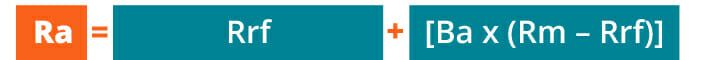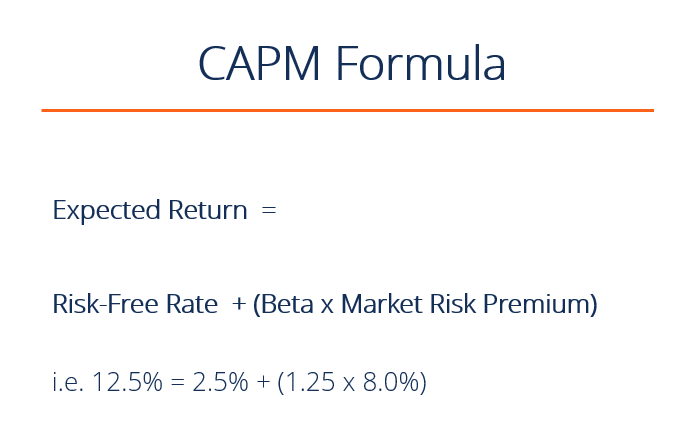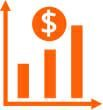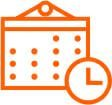# Capital Asset Pricing Model (CAPM)

A method for calculating the required rate of return, discount rate or cost of capital

## What is CAPM?

The Capital Asset Pricing Model (CAPM) is a model that describes the relationship between the expected return and risk of investing in a security. It shows that the expected return on a security is equal to the risk-free return plus a risk premium, which is based on the beta of that security. Below is an illustration of the CAPM concept.Image: CFI’s Math for Corporate Finance Course.

### CAPM Formula and Calculation

CAPM is calculated according to the following formula:

#####Where:

Ra = Expected return on a security
Rrf = Risk-free rate
Ba = Beta of the security
Rm = Expected return of the market

Note: “Risk Premium” = (Rm – Rrf)

The CAPM formula is used for calculating the expected returns of an asset.  It is based on the idea of systematic risk (otherwise known as or non-diversifiable risk) and that investors need to be compensated for it in the form of a risk premium. A risk premium is a rate of return greater than the risk-free rate. When investing, investors desire a higher risk premium when taking on more risky investments.### Expected ReturnThe “Ra” notation above represents the expected return of a capital asset over time, given all of the other variables in the equation.  “Expected return” is a long-term assumption about how an investment will play out over its entire life.

### Risk-Free RateThe “Rrf” notation is for the risk-free rate, which is typically equal to the yield on a 10-year US government bond.  The risk-free rate should correspond to the country where the investment is being made, and the maturity of the bond should match the time horizon of the investment. Professional convention, however, is to typically use the 10-year rate no matter what, because it’s the most heavily quoted and most liquid bond.

### BetaThe beta (denoted as “Ba” in the CAPM formula) is a measure of a stock’s risk (volatility of returns) reflected by measuring the fluctuation of its price changes relative to the overall market. In other words, it is the stock’s sensitivity to market risk. For instance, if a company’s beta is equal to 1.5 the security has 150% of the volatility of the market average. However, if the beta is equal to 1, the expected return on a security is equal to the average market return.  A beta of -1 means security has a perfect negative correlation with the market.

From the above components of CAPM, we can simplify the formula to reduce “expected return of the market minus the risk-free rate” to be simply the “market risk premium”.  The market risk premium represents the additional return over and above the risk-free rate, which is required to compensate investors for investing in a riskier asset class. Put another way, the more volatile a market or an asset class is, the higher the market risk premium will be.

### Video Explanation of CAPM

Below is a short video explanation of how the Capital Asset Pricing Model works and why it’s important for financial modeling and valuation in corporate finance. To learn more, check out CFI’s Financial Analyst Courses.

### Why CAPM is Important

The CAPM formula is widely used in the finance industry. It is vital in calculating the weighted average cost of capital (WACC) as CAPM computes the cost of equity.

WACC is used extensively in financial modeling.  It can be used to find the net present value (NPV) of the future cash flows of an investment and to further calculate its enterprise value and finally its equity value.

### CAPM Example – Calculation of Expected Return

Let’s calculate the expected return on a stock, using the Capital Asset Pricing Model (CAPM) formula. Suppose the following information about a stock is known:

• It trades on the NYSE and its operations are based in the United States
• Current yield on a U.S. 10-year treasury is 2.5%
• The average excess historical annual return for U.S. stocks is 7.5%
• The beta of the stock is 1.25 (meaning it’s average weekly return is 1.25x as volatile as the S&P500 over the last 2 years)

What is the expected return of the security using the CAPM formula?

Let’s break down the answer using the formula from above in the article:

• Expected return = Risk Free Rate + [Beta x Market Return Premium]
• Expected return = 2.5% + [1.25 x 7.5%]
• Expected return = 11.9%

### More Resources

We hope this CFI guide to the CAPM formula has been helpful.  To continue learning and advancing your finance career as a financial analyst, we have developed several more articles that are highly relevant.  To learn more, please see:

• WACC
• Asset beta
• Valuation methods
• Comparable company analysis
• Financial modeling guide

### Financial Analyst Training

Get world-class financial training with CFI’s online certified financial analyst training program!

Gain the confidence you need to move up the ladder in a high powered corporate finance career path.

Learn financial modeling and valuation in Excel the easy way, with step-by-step training.